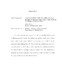## Calculations for the etale fundamental group of curves of genus one or two

 dc.contributor.advisor Ramachandran, Niranjan en_US dc.contributor.author Lizzi, Adam Joseph en_US dc.contributor.department Mathematics en_US dc.contributor.publisher Digital Repository at the University of Maryland en_US dc.contributor.publisher University of Maryland (College Park, Md.) en_US dc.date.accessioned 2018-09-07T05:32:56Z dc.date.available 2018-09-07T05:32:56Z dc.date.issued 2018 en_US dc.description.abstract The \'etale fundamental group $\pi_{1, \text{\'et}}(C)$ is a profinite group which classifies covers of algebraic-geometric objects. Its abelianizaton classifies torsors over $C$ with a finite, abelian structure group. When $C$ is a curve over a number field $K$, $\pi_{1, \text{\'et}}^{\textsf{ab}}(C)$ possesses a finite subgroup $\Ker(C/K)$ which records which abelian \'etale covers of $C$ come from geometry. In this thesis multiple algorithms for calculating $\Ker(E/K)$ are presented when $E$ is an elliptic curve over a number field. We indicate how to generalize one of these algorithms to the Jacobian of a genus two curve $C$, allowing for progress to be made on calculating $\Ker(C/K)$. en_US dc.identifier https://doi.org/10.13016/M2TB0XZ7N dc.identifier.uri http://hdl.handle.net/1903/21123 dc.language.iso en en_US dc.subject.pqcontrolled Mathematics en_US dc.subject.pquncontrolled elliptic curves en_US dc.subject.pquncontrolled etale fundamental group en_US dc.subject.pquncontrolled jacobian en_US dc.subject.pquncontrolled number theory en_US dc.title Calculations for the etale fundamental group of curves of genus one or two en_US dc.type Dissertation en_US
##### Original bundle
Now showing 1 - 1 of 1